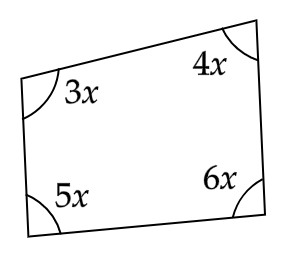# The angles of a quadrilateral are in the ratio $3:4:5:6$. How do you find the angles of the quadrilateral?Verified
96.9k+ views
Hint: Given, the four angles of a quadrilateral are in the ratio $3:4:5:6$. We will assume the four angles to be $3x$, $4x$, $5x$ and $6x$ respectively. Using the angle sum property of a quadrilateral we form an equation in terms of $x$. We will solve the equation to find the value of $x$ and we will use the value of $x$ to find the measure of the four angles of the quadrilateral.It is given that the angles of the quadrilateral are in the ratio $3:4:5:6$.
Let the four angles of the given quadrilateral be $3x$, $4x$, $5x$ and $6x$ respectively.
Now, from the angle sum property of a quadrilateral we know that the sum of the measures of the four interior angles of a quadrilateral is always ${360^ \circ }$.
Using this, we can write
$\Rightarrow 3x + 4x + 5x + 6x = {360^ \circ }$
Adding the like terms in the above equation, we get
$\Rightarrow 18x = {360^ \circ }$
Dividing both the sides of the equation by $18$, we get
$\Rightarrow \dfrac{{18x}}{{18}} = \dfrac{{{{360}^ \circ }}}{{18}}$
Cancelling the common terms from the numerator and the denominator, we get
$\Rightarrow x = {20^ \circ }$
Finally, we will substitute the value of $x$ to find the measures of all the angles of the quadrilateral.
On doing this, we get the four angles of the given quadrilateral as $\left( {3 \times {{20}^ \circ }} \right)$, $\left( {4 \times {{20}^ \circ }} \right)$, $\left( {5 \times {{20}^ \circ }} \right)$ and $\left( {6 \times {{20}^ \circ }} \right)$ i.e., ${60^ \circ }$, ${80^ \circ }$, ${100^ \circ }$ and ${120^ \circ }$ respectively.
Therefore, the angles of the quadrilateral are ${60^ \circ }$, ${80^ \circ }$, ${100^ \circ }$ and ${120^ \circ }$.

Note: Here, the question is of a quadrilateral. Like the sum of the measure of the angles of a quadrilateral is always ${360^ \circ }$. Similarly, the sum of the measure of the angles of a triangle having three sides is always ${180^ \circ }$, the sum of the measure of the angles of a pentagon having five sides is always ${540^ \circ }$ and the sum of the measure of the angles of a hexagon having six sides is always ${720^ \circ }$.